Like   Tweet   Pin   in

# MARCH 2020

 /* styles */ Dear Friend,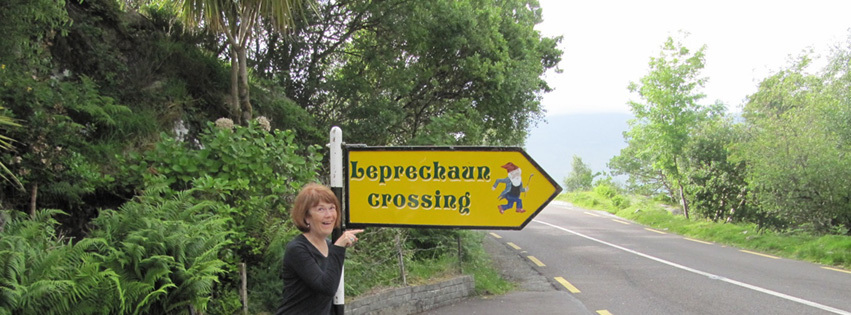/* styles */ A very happy St. Patrick's Day to you and your loved ones this month! I so look forward to this time of year and to celebrating the leprechauns and other elementals in my life. Celebrate with me by taking advantage of our sale on leprechaun books and DVD for the month of March. There are still seats left in the Iceland Magical Mystical tour in May, and my Canadian workshop on working with nature spirits this month. Summer activities are fast approaching with dates in Europe and our annual retreat here at Diamond Heart, in BC, Canada. Enjoy your St. Patrick's Day and our March month of celebrating all things elemental ("...an' especially us leprechauns"--Lloyd)!
 table div table+table+table+table+table+table div table{width:100%;padding:0}table div table+table+table+table+table+table div table img{width:96.23%;padding:0;float:none}table div table+table+table+table+table+table div table td{width:100%;padding:0 1.88% 18px}/* styles *//* styles */ “Send energy to elementals who look after trees, flowers, water, and mountains, to keep them healthy. Do this with joy and gratitude.” --Tanis Helliwell, from Summer with the Leprechauns
 table div table+table+table+table+table+table+table+table div table{width:100%;padding:0}table div table+table+table+table+table+table+table+table div table img{width:96.23%;padding:0;float:none}table div table+table+table+table+table+table+table+table div table td{width:100%;padding:0 1.88% 18px}/* styles */## ARTICLE: Leprechauns and the 'Craic'

 /* styles */ by Lloyd the Leprechaun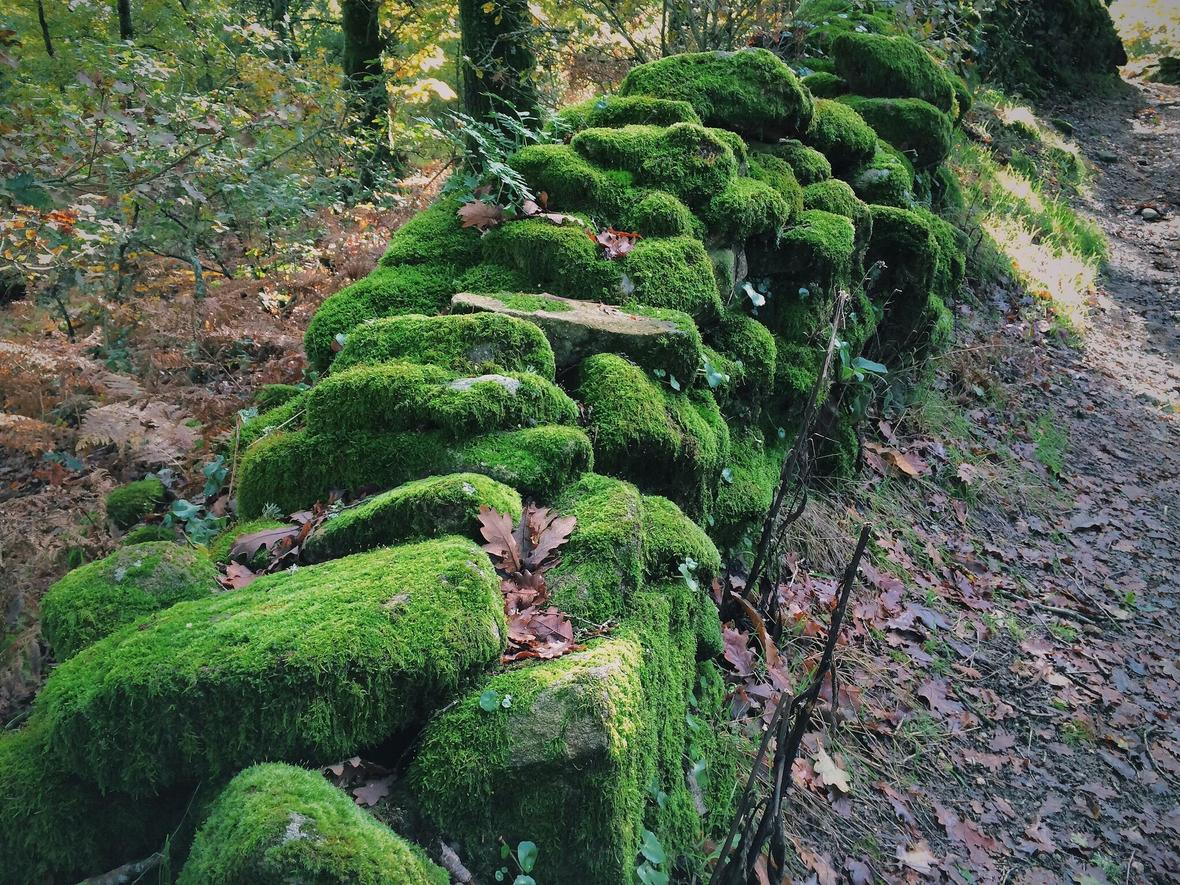/* styles */ Hello - Lloyd the leprechaun here! If you’re not Irish, you’ve probably never heard of the Craic. Well that’s something very important you’ve been missing. The truth is that leprechauns taught the Irish about the Craic. It basically means to keep your sense of humor, have a good time, and don’t get your knickers tied in a knot when things aren’t going the way you thought they should. Humans do so love to control their life, but what’s the fun in having everything all predictable. No surprises. BORING! Even elves have a tendency to take themselves too seriously, like to look good to others, that sort of thing. So leprechauns regard it as their sacred duty to help those poor elves and humans to lighten up by turning their expectations bottom side up. I did this with a tour that Tanis led a group of 30 pilgrim’s on — she wrote about it in Pilgrimage with the Leprechauns and if you’d like to hear more just read along.
 table div table+table+table+table+table+table+table+table+table+table+table+table+table+table div table{width:100%;padding:0}table div table+table+table+table+table+table+table+table+table+table+table+table+table+table div table img{width:96.23%;padding:0;float:none}table div table+table+table+table+table+table+table+table+table+table+table+table+table+table div table td{width:100%;padding:0 1.88% 18px}/* styles */# BOOKS:

Order during the month of March to get your 10% discount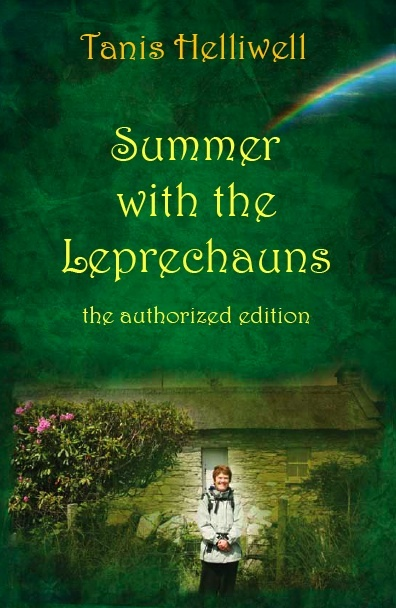AN IRISH JEWEL, far more than a fairy tale. A good humored, true account of Tanis’ summer in a cottage in Ireland where the resident leprechaun taught her about the evolution of elementals (fairies, elves, leprechauns). Learn about the importance of humans and elementals working together for the betterment of both of their races and the Earth.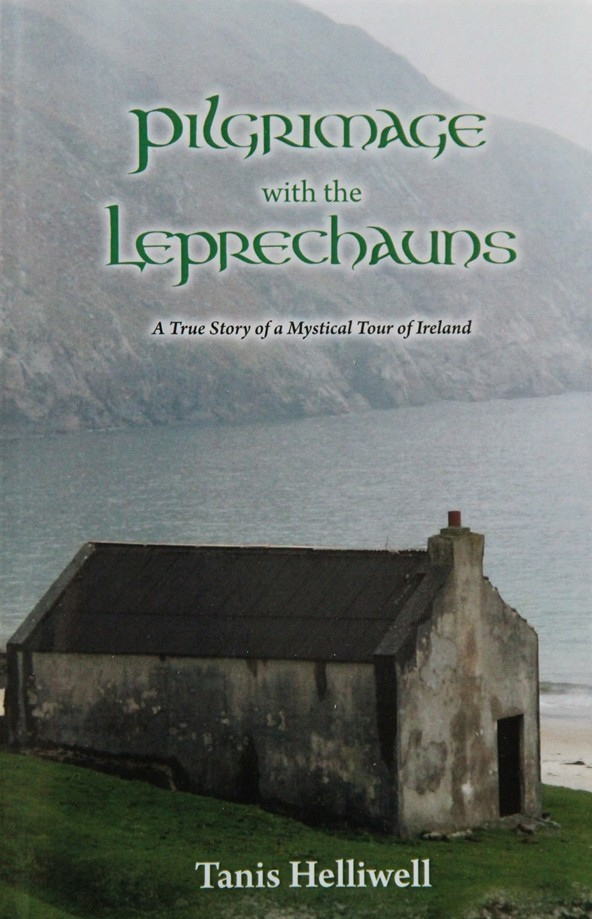FULL OF INFORMATION about nature spirits or elementals, as they like to call themselves. The trolls, elves, goblins, and brownies are as real for the reader as the various humans whom they partner. For anyone planning a trip to the sacred sites of Ireland, or to Ireland itself for that matter, this book abounds in nuggets as precious as the pot of gold that the Leprechaun holds at the end of the rainbow.
 table div table+table+table+table+table+table+table+table+table+table+table+table+table+table+table+table+table+table+table div table{width:100%;padding:0}table div table+table+table+table+table+table+table+table+table+table+table+table+table+table+table+table+table+table+table div table img{width:96.23%;padding:0;float:none}table div table+table+table+table+table+table+table+table+table+table+table+table+table+table+table+table+table+table+table div table td{width:100%;padding:0 1.88% 18px}/* styles */table div table+table+table+table+table+table+table+table+table+table+table+table+table+table+table+table+table+table+table+table+table div table{width:100%;padding:0}table div table+table+table+table+table+table+table+table+table+table+table+table+table+table+table+table+table+table+table+table+table div table img{width:96.23%;padding:0;float:none}table div table+table+table+table+table+table+table+table+table+table+table+table+table+table+table+table+table+table+table+table+table div table td{width:100%;padding:0 1.88% 18px}/* styles */# Elementals DVD:

 table.module-22{width:95.09%;padding:0}table div table+table+table+table+table+table+table+table+table+table+table+table+table+table+table+table+table+table+table+table+table+table+table div table{width:95.09%;float:none;margin-left:auto;margin-right:auto;padding:0}table div table+table+table+table+table+table+table+table+table+table+table+table+table+table+table+table+table+table+table+table+table+table+table div table a{border:0 none;text-decoration:none}table div table+table+table+table+table+table+table+table+table+table+table+table+table+table+table+table+table+table+table+table+table+table+table div table img{width:100%!important;border:0 none;text-decoration:none}table div table+table+table+table+table+table+table+table+table+table+table+table+table+table+table+table+table+table+table+table+table+table+table div table td{width:100%;padding:0}/* styles */
 /* styles */ Learn to work and play with elementals, also called nature spirits, in this informative and engaging DVD with real images of elementals. With the good-humoured spirit akin to an Irish story-teller Tanis brings us on a journey to learn more about elementals. You will come away from this DVD with: * an appreciation of the beings with whom humans share this planet * tools to work with the elementals * an understanding of why it is essential that humans learn to co-create with natural and spiritual laws in order to become guardians of the Earth This timely DVD assists us to more fully embrace all consciousness on Earth and to take responsibility for our place in the plan.
 table div table+table+table+table+table+table+table+table+table+table+table+table+table+table+table+table+table+table+table+table+table+table+table+table+table+table div table{width:100%;padding:0}table div table+table+table+table+table+table+table+table+table+table+table+table+table+table+table+table+table+table+table+table+table+table+table+table+table+table div table img{width:96.23%;padding:0;float:none}table div table+table+table+table+table+table+table+table+table+table+table+table+table+table+table+table+table+table+table+table+table+table+table+table+table+table div table td{width:100%;padding:0 1.88% 18px}/* styles */# March 13, 14 & 15 | Victoria, BC Canada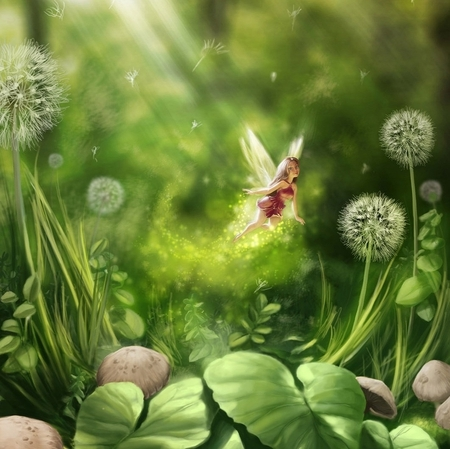/* styles */ March 13, 2020 - TALK & BOOK SIGNING: Why Working with Nature Spirits and Ancestors are Essential Today! Church of Truth, Victoria, http://cotvictoria.ca/ Time: 7:30-9:30pm Fee: \$20 at door Contact: Iris Scruton, wangchiaoyin(at)gmail.com Phone: +1-250-889-0042 March 14/15, 2020 - WORKSHOP: Humans to Work & Play with Nature Spirits to HEAL the EARTH Place: McTavish Academy of Art (https://mctavishacademy.ca/), 1720 McTavish Rd, North Saanich, BC Time: 9:30am to 5pm Saturday and 9:30 to 4 on Sunday Fee: \$245. Participants bring their own lunch for Saturday and bring a dish to share for Sunday potluck lunch. Tea, coffee, and snacks are provided during AM/PM breaks. Contact: Iris Scruton, wangchiaoyin(at)gmail.com Phone: +1-250-889-0042 To register for the workshop send payment by E-transfer to wangchiaoyin(at)gmail.com
 table div table+table+table+table+table+table+table+table+table+table+table+table+table+table+table+table+table+table+table+table+table+table+table+table+table+table+table+table+table+table+table div table{width:100%;padding:0}table div table+table+table+table+table+table+table+table+table+table+table+table+table+table+table+table+table+table+table+table+table+table+table+table+table+table+table+table+table+table+table div table img{width:96.23%;padding:0;float:none}table div table+table+table+table+table+table+table+table+table+table+table+table+table+table+table+table+table+table+table+table+table+table+table+table+table+table+table+table+table+table+table div table td{width:100%;padding:0 1.88% 18px}/* styles */## Pssst!

 /* styles */ The annual Diamond Heart Meditation Retreat is fast approaching, July 19-25, 2020 on the Sunshine Coast, Canada. Register before May 31 and save \$100!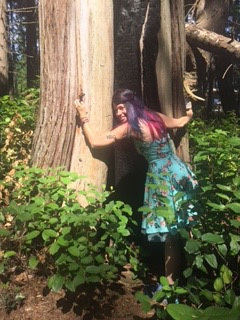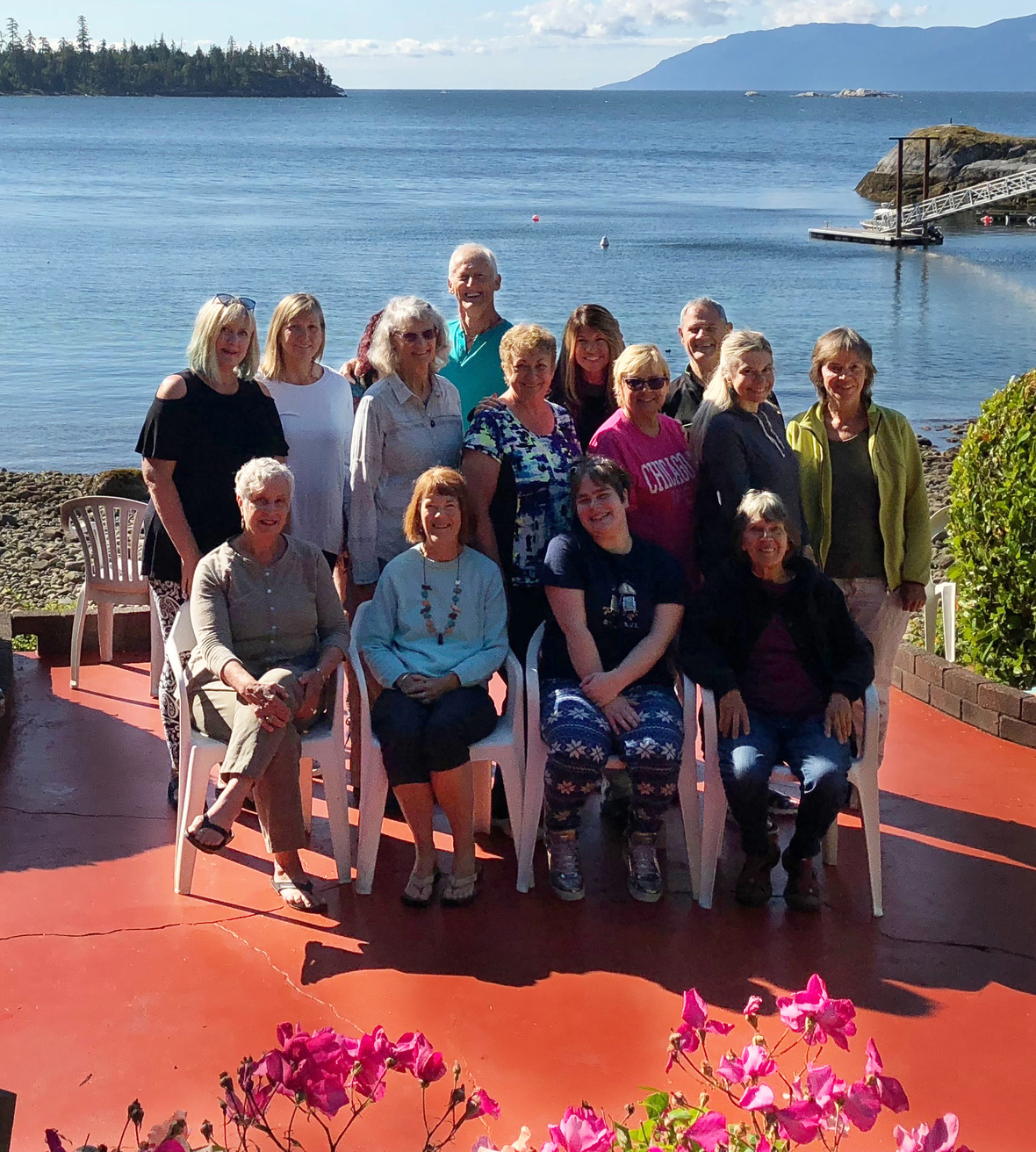/* styles */
 table div table+table+table+table+table+table+table+table+table+table+table+table+table+table+table+table+table+table+table+table+table+table+table+table+table+table+table+table+table+table+table+table+table+table+table+table+table div table{width:100%;padding:0}table div table+table+table+table+table+table+table+table+table+table+table+table+table+table+table+table+table+table+table+table+table+table+table+table+table+table+table+table+table+table+table+table+table+table+table+table+table div table img{width:96.23%;padding:0;float:none}table div table+table+table+table+table+table+table+table+table+table+table+table+table+table+table+table+table+table+table+table+table+table+table+table+table+table+table+table+table+table+table+table+table+table+table+table+table div table td{width:100%;padding:0 1.88% 18px}/* styles */# 2 seats left for Iceland tour!Two more seats are now available for the Magical Mystical Tour of Iceland!
 table div table+table+table+table+table+table+table+table+table+table+table+table+table+table+table+table+table+table+table+table+table+table+table+table+table+table+table+table+table+table+table+table+table+table+table+table+table+table+table+table+table+table div table{width:100%;padding:0}table div table+table+table+table+table+table+table+table+table+table+table+table+table+table+table+table+table+table+table+table+table+table+table+table+table+table+table+table+table+table+table+table+table+table+table+table+table+table+table+table+table+table div table img{width:96.23%;padding:0;float:none}table div table+table+table+table+table+table+table+table+table+table+table+table+table+table+table+table+table+table+table+table+table+table+table+table+table+table+table+table+table+table+table+table+table+table+table+table+table+table+table+table+table+table div table td{width:100%;padding:0 1.88% 18px}/* styles */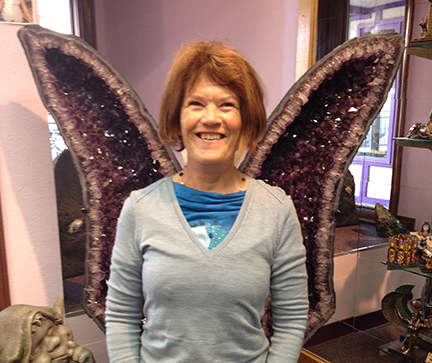table div table+table+table+table+table+table+table+table+table+table+table+table+table+table+table+table+table+table+table+table+table+table+table+table+table+table+table+table+table+table+table+table+table+table+table+table+table+table+table+table+table+table+table+table+table+table div table{width:100%;padding:0}table div table+table+table+table+table+table+table+table+table+table+table+table+table+table+table+table+table+table+table+table+table+table+table+table+table+table+table+table+table+table+table+table+table+table+table+table+table+table+table+table+table+table+table+table+table+table div table img{width:96.23%;padding:0;float:none}table div table+table+table+table+table+table+table+table+table+table+table+table+table+table+table+table+table+table+table+table+table+table+table+table+table+table+table+table+table+table+table+table+table+table+table+table+table+table+table+table+table+table+table+table+table+table div table td{width:100%;padding:0 1.88% 18px}/* styles */